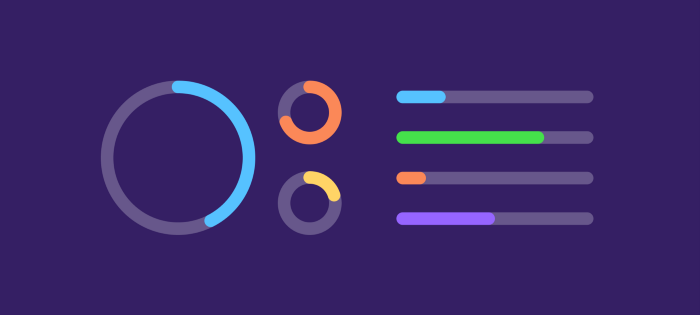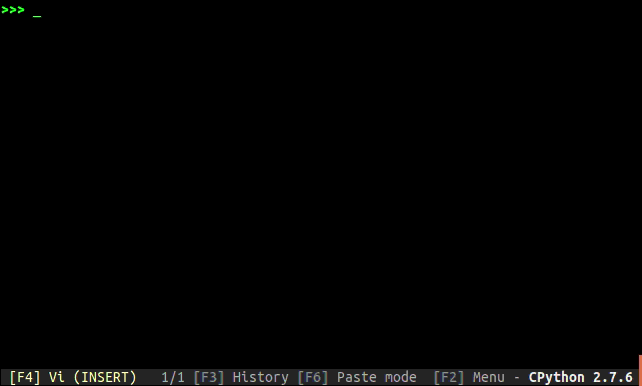When using Python to perform time-consuming operations, it is common to use a progress bar to visualize the progress. tqdm in Python is used to achieve this function.First, let’s see the effect of tqdm’s progress bar.## Basic tqdm usage

There are 3 main uses of tqdm, automatic control, manual control or for scripting or command line.

Automatically controlled operation

The most basic usage, wrapping tqdm() directly around an arbitrary iterator.

 ``````1 2 3 4 5 6 7 `````` ``````from tqdm import tqdm import time text = "" for char in tqdm(["a", "b", "c", "d"]): text = text + char time.sleep(0.5) ``````

trange(i) is a specially optimized instance of tqdm(range(i)).

 ``````1 2 3 4 5 `````` ``````from tqdm import trange import time for i in trange(100): time.sleep(0.1) ``````

If instantiated outside the loop, manual control of tqdm() can be allowed.

 ``````1 2 3 4 5 6 7 `````` ``````from tqdm import tqdm import time pbar = tqdm(["a", "b", "c", "d"]) for char in pbar: pbar.set_description("Processing %s" % char) time.sleep(0.5) ``````

Manual control of the run

Manually control the update of tqdm() with the with statement.

 ``````1 2 3 4 5 6 7 `````` ``````from tqdm import tqdm import time with tqdm(total=100) as pbar: for i in range(10): pbar.update(10) time.sleep(0.5) ``````

Or without the with statement, but with the del or close() method at the end.

 ``````1 2 3 4 5 6 7 8 `````` ``````from tqdm import tqdm import time pbar = tqdm(total=100) for i in range(10): pbar.update(10) time.sleep(0.5) pbar.close() ``````

The tqdm.update() method is used to manually update the progress bar and is useful for streaming operations such as reading files.

 `````` 1 2 3 4 5 6 7 8 9 10 11 12 `````` ``````from multiprocessing import Pool import tqdm import time def _foo(my_number): square = my_number * my_number time.sleep(1) return square if __name__ == '__main__': with Pool(2) as p: r = list(tqdm.tqdm(p.imap(_foo, range(30)), total=30)) ``````